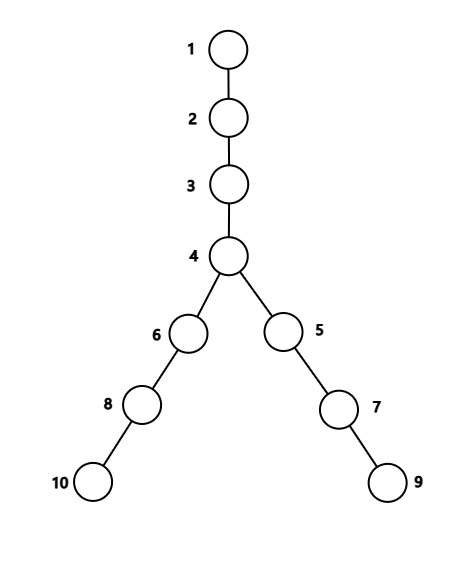# UOJ1. 填的数都是非负整数。
2. 对于第 $i(2\leq i)$ 个节点，它父亲填的数必须不小于它。
3. 所填数之和恰好为 $n$。

### 样例一

#### input

9 4



#### output

1 2 3 5 8 14 22 36 56



#### explanation

1. $$
2. $[4,1]$
3. $[3,2]$
4. $[3,1,1]$
5. $[2,2,1]$
6. $[2,1,1,1]$
7. $[1,1,1,1,1]$
8. $[1,1,1,1,0,1]$

### 数据范围与提示

$1$ $100$ $20$ $10$
$2$ $1000$ $100$ $20$
$3$ $500000$ $1$ $10$
$4$ $2$ $30$
$5$ $500$ $30$